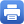Columbia Home
Algebraic Topology

This course is taken in sequence, part 1 in the fall, and part 2 in the spring.

### Algebraic Topology I

I Homology Theory

• Singular homology — definition, simple computations
• Cellular homology — definition
• Eilenberg-Steenrod Axioms for homology
• Computations: Sn, RPn, CPn, Tn, S2^S3, Grassmannians, X*Y
• Alexander duality — Jordan curve theorem and higher dimensional analogues
• Applications: Winding number, degree of maps, Brouwer fixed point theorem
• Lefschetz fixed point theorem

II Homotopy Theory

• Homotopy of maps, of pointed maps
• The homotopy category and homotopy functors –examples
• π1(X, x0)
• Van Kampen’s theorem
• Higher homotopy groups and the Hurewicz theorem
• π3(S2)
• Higher homotopy groups of the sphere

III Covering Spaces

• Definition of a covering projection
• Examples — Coverings of S1, Sn covering RPn, Spin(n) covering SO(n)
• Homotopy path lifting
• Classification of coverings of a reasonable space

IV Homology with Local Coefficients

• Local coefficient systems
• Relation with covering spaces
• Obstruction theory
• The Alexander polynomial of a knot

### Algebraic Topology II

I Cohomology

• Cup products
• Pairings homology
• Cohomology and homology with coefficients
• Universal coefficient theorems

II Cech Cohomology

• Open coverings and Cech cochains
• The coboundary mapping
• Cech cohomology
• Comparison with singular cohomology

III Selected Topics

• Group Cohomology
• Sheaf Cohomology
• de Rham’s theorem
• Morse functions and Poincaré duality for manifolds
• Thom Isomorphism Theorem and cohomology classes Poincaré dual to cyclesPrint this page